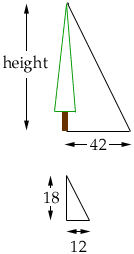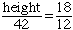a tree's shadow is 42 ft. long. There is a stop sign that is right next to it and it is 18 ft. tall and it's shadow is 12 ft. long. How tall is the tree? How would i figure out this problem? Hi Ronda, First draw a diagram.The shadow in each case is created by the object, (tree or sign) blocking the light from the sun. Since the angle which which the sun's rays strke the tree and the sign are the same, the triangles in the diagram are similar. HenceCheers, Penny Go to Math Central